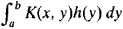# Eigenfunction

(redirected from Eigenfunctions)
Also found in: Dictionary, Medical.

## Eigenfunction

One of the solutions of an eigenvalue equation. A parameter-dependent equation that possesses nonvanishing solutions only for particular values (eigenvalues) of the parameter is an eigenvalue equation, the associated solutions being the eigenfunctions (sometimes eigenvectors). In older usage the terms characteristic equation and characteristic values (functions) are common. Eigenvalue equations appear in many contexts, including the solution of systems of linear algebraic equations (matrix equations), differential or partial differential equations, and integral equations. The importance of eigenfunctions and eigenvalues in applied mathematics results from the widespread applicability of linear equations as exact or approximate descriptions of physical systems. However, the most fundamental application of these concepts is in quantum mechanics where they enter into the definition and physical interpretation of the theory. Only linear eigenvalue equations will be discussed. See Eigenvalue (quantum mechanics), Energy level (quantum mechanics), Quantum mechanics

## Eigenfunction

a concept of mathematical analysis. The solution of many problems in mathematical physics—for example, in the theory of vibrations and the theory of heat conduction—requires the finding of nontrivial solutions of homogeneous linear differential equations L(y) = Xy where the solutions satisfy particular boundary conditions. Such solutions are called ei-genfunctions of the problem, and the corresponding values of λ are called eigenvalues.

If a differential equation with appropriate boundary conditions is self-adjoint, then its eigenvalues are real, and the eigenfunctions corresponding to distinct eigenvalues are orthogonal. If the differential equation is considered on a finite interval and its coefficients have no singularities on the interval, then the set of eigen-functions is countable—that is, the problem has a discrete spectrum. If we know the eigenfunctions and the corresponding eigenvalues, then, under certain conditions, we can obtain a solution of the problem in the form of a series of eigenfunctions. Suppose, however, the equation is considered on an infinite interval or its coefficients have singularities (for example, the coefficient of the derivative of highest order takes on the value 0). A continuum of eigenfunctions may then exist, and expansion in the form of an integral with respect to the eigenfunctions is obtained instead of a series expansion. Such an integral is analogous to a Fourier integral representation. In this case, the problem is said to have a continuous spectrum. Many special functions, such as orthogonal polynomials, serve as the eigenfunctions of some equations.

In the theory of integral equations, a function that for some value of X satisfies the equationis called an eigenfunction of the kernel K(x, y). Any symmetric continuous kernel has an eigenfunction. In this case, any function representable in the formcan be expanded in a series of eigenfunctions. If the kernel has singularities or is defined in an infinite domain, then a continuous spectrum may also arise.

Eigenfunctions can be defined most generally as the eigenvectors of linear operators in linear function spaces. In quantum mechanics, the eigenfunctions of an operator corresponding to some physical quantity correspond to those states of a system in which the given physical quantity has a definite value.

Eigenfunctions are sometimes also called proper functions or characteristic functions.

## eigenfunction

[′ī·gən‚fəŋk·shən]
(mathematics)
Also known as characteristic function.
An eigenvector for a linear operator on a vector space whose vectors are functions. Also known as proper function.
A solution to the Sturm-Liouville partial differential equation.
References in periodicals archive ?
I found this way of treating the degeneration rather convenient, since it gives a simple way of finding two independent eigenfunctions for each set of energy and momentum".
It is straightforward to show that the eigenfunctions {[[PSI].
In spherical polar coordinates, amplitude functions [psi](r,[theta],[phi]) are not eigenfunctions of operator A; its component [A.
The exact eigenvalues and eigenfunctions are well known and easily derived.
Yao, Completeness of eigenfunctions of Sturm-Liouville problems with transmission conditions, Methods Appl.
Note that different eigenvalues can have the same eigenfunction as the eigenfunctions do not depend on l.
A solution of differential equation of motion was assumed as a product of eigenfunctions of displacement and time [2, 3]:
Because region 2 has the same cross-sectional dimensions as region 1, the radial eigenfunctions of the two regions are the same for the TEM mode
The solution thus obtained is a sum of triple quadratures which expressed in terms of eigenfunctions and eigenvalues of corresponding vertical spectral problems.
The study of this structure, using the rigorous derivation of the vector eigenfunctions of a parallel plates waveguide, has been presented in [9,10].
In this technique, the dimension of the linear equations system is determined from the number of eigenfunctions used in the series expansion.

Site: Follow: Share:
Open / Close